Green functions in R<sup>n</sup>.

## Linear Methods of Applied Mathematics Evans M. Harrell II and James V. Herod*

version of 21 February 1999

## Green functions in Rn.

The printed version of these notes is an appendix to the rest. In this Web version, it consists of links bringing out the analogies between integral operators and matrices.

The common setting for the two subjects is an inner product space, which was defined abstractly in the chapter entitled The Geometry of Functions. Since the idea of an inner product, or dot product, arises in such a variety of problems, we should recall exactly what are the properties that define an inner product and what are some of the consequences of these properties.

Appendix A1 ARITHMETIC AND GEOMETRY IN Rn

If E is the linear space on which the inner product is defined and x and y are in E, then < x, y > denotes the (perhaps, complex) number which is the inner product of x and y. Moreover

(a) <x,y> = <y,x>* for all x,y in ExE (Note that * is the complex conjugate),

(b) < [[alpha]]x+y, z > = [[alpha]]< x, z > + < y, z > for all numbers [[alpha]] and all x and y in E,

(c) < x, x > > 0 if x !=0, with < x, x > = 0 if x = 0.

One consequence of these defining properties is the Cauchy-Schwartz inequality: if x and y are in E then

|< x, y >|2 <= < x, x > < y, y >.

A norm is defined in terms of the inner product:

||x||2 = < x , y >.

This norm has those properties which characterize any norm:

||x|| > 0 unless x = 0,

||ax|| = |a| ||x||, and

||x+y|| <= ||x|| + ||y||.

EXAMPLE: One can think of a weighted dot product on Rn by choosing a positive number sequence {ap}O(p=1,n) and defining < x, y > to be given by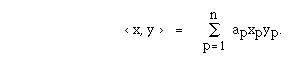The usual inner product on Rn is the one obtained in the above example by choosing ap = 1 for p= 1,2,...n. In this setting, we can think of the structure of Rn in the language of Euclidean geometry: the distance between x and y is ||x-y|| and the angle between x and y is [[theta]] where 0 <= [[theta]] <= [[pi]] and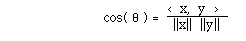provided neither x nor y is zero. For example, points x and y are perpendicular, or orthogonal, if < x, y > = 0. Also, the concept of distance provides a notion of points being "close together". It is natural to say that the sequence {vp} of points in Rn has limit the point y in Rn provided

limp || vp - y || = 0.

It is of value, even at this elementary level, to realize that there are several ways to think of the idea "limp vp = y". In Rn, the following three are equivalent:

The sequence {vp} in Rn converges to y component wise if for each integer i, 1 <= i <= n,

limp(vp)i = yi.

The sequence {vp} in Rn converges to y uniformly if

limp( maxi | xp(i) -y(i) | )= 0.

The sequence {vp} in Rn converges to y in norm if

limp||vp - y|| =0.

It is not difficult to establish that these three notions are equivalent in Rn. The value of thinking of them separately here is that the three methods of convergence have analogues in situations which we will encounter in later sections. In those situations, the three methods of convergence may be not equivalent.

Fundamental in the development of Green's Functions will be the Riesz Representation Theorem: If L is a linear function from Rn to R then there is a vector v in Rn such that L(u) = < u, v > for all u in Rn. Closely related to the Riesz Representation Theorem is the fact that every linear function from Rn to Rn has a matrix representation. These ideas are familiar.

Linear functions and matrices arise in many ways. Here is one that you might not have considered. Choose an nxn matrix A. Consider all points x and y in Rn related by

< Au, x > = < u, y >

for all u in Rn. To be sure that, given x, there is such a point y, consider the following: pick x; then L(u) = <Au, x > is a linear function of u from Rn to R. By the Riesz Representation Theorem, there is a point y in Rn such that L(u) = < u, y > for all u in Rn. Define this y as B(x). It can be established that B is, itself, a linear function from Rn to Rn. Hence, it has a matrix representation. This linear function B is called the adjoint of A and is denoted as A*. For all x and u in Rn,

< Au, x > = < u, A*x >.

The concern of this course, stated in the context of Rn, is the following problem: given a matrix A and a vector f = {f1 ,f2 , ...fn}, can a vector u be found such that Au = f? There are matrices A and vectors f such that the equation Au=f has exactly one solution, or no solution, or an infinity of solutions.

Appendix A3 THE FREDHOLM ALTERNATIVE THEOREMS

The following ideas will persist in each segment of the course. These fundamental results are known as the Fredholm Alternative Theorems. For matrices, the alternatives hinge on whether or not the determinant of A is zero or not.

I. Exactly one of the following two alternatives holds:

(a)(First Alternative) if f is in Rn, then Au = f has one and only one solution.

(b)(Second Alternative) Au= 0 has a nontrivial solution.

II. (a) If the first alternative holds for A, then it also holds for A*.

(b) In either alternative, the equations Au= 0 and A* u = 0 have the same number of linearly independent solutions.

III. Suppose the second alternative holds. Then Au=f has a solution if and only if < f, z > = 0 for each z such that A*z=0.

A matrix problem is well- posed if

(a) for each f, the equation Au=f has a solution,

(b) the solution is unique, and

(c) the solution depends continuously on the data-- in the sense that if f is close to g and u and v satisfy Au=f and Av = g, then u is close to v.

Appendix A4 SOLVING EQUATIONS

Just as there are many ways to conceive of solving Au=f in case A is a matrix, so there are many ways for finding solutions for problems which are introduced in the next chapters. We concentrate on the method of constructing a Green's function. From what has come before, it may be clear that in some sense, we are finding an inverse for the linear operation A.

THE FIRST ALTERNATIVE

We discuss the solution of the linear equation

Au = f,

where we suppose we are given a matrix A and know f. Don't be to quick to dismiss the solution as u = A-1 f. While that is correct, we want perspective here, not just results.

Here is an adaptation of the methods which we will use to find Green's functions in two chapters from here: Let deltai be the vector which has the property that

< deltai, u > = u(i)

for all u in Rn. One can write the components for deltai

deltai = {0,0,...0. 1, 0, ... 0}

where the 1 is in the i-th component. Note that

u = [[Sigma]]i deltai u(i).

Let A be an nxn matrix and f be a vector. In order to solve Au=f, we seek G(i,j) such that u defined by

< G(j,.) , f(.) > = u(j) (*)

might provide the solution for Au=f.

Look again at equation (*). Writing the dot product as a sum changes that equation toor, in the notation of matrix multiplication,

Gf = u.

In what follows, perhaps you will see that writing the equation as (*) or (**) provides unifying ideas.

Here is a proposal for how humans find G. Find G such that

A(G( ,i))= deltai.

That is, A(G( ,i))(m) = deltai(m).

Having such a G, define u by

u(j) = [[Sigma]] i G(j,i)f(i).

Then

[A(u)](m) = [[Sigma]]j A(m,j) u(j) = [[Sigma]] j A(m,j) [[Sigma]] i G(j,i)f(i)

= [[Sigma]] i [[Sigma]] j A(m,j)G(j,i)f(i)

= [[Sigma]] ideltai(m) f(i) = f(m).

This is what was desired: u solves the equation

Au = f.

Here is an alternate approach to the problem: Consider these relations:

<deltai,u> = u(i) = <G(i,.),f(.)> = <G(i,.),Au> = <A*G(i,.),u>.

Thus, we might seek G such that deltai = A*G(i,.). Go back and re-do the above exercise this way to see if you get the same answer. Here's a proof that you should fill in with details.

THEOREM. Suppose that A and G are matrices. These are equivalent:

(a) A(G( ,i)) = deltai and (b) A*(G(i, )) = deltai.

Suggestion for a proof. Let G1 be defined by the first equation:

A(G1( ,i)) = deltai

and G2 be defined by the second equation:

A*(G2(i, )) = deltai.

Then G1(j,i) = < dj , G1( ,i) > = < A*(G2(j, )) , G1( ,i)) >

= < G2(j, ) , A(G1( ,i)) > = < G2(j, ) , deltai > = G2(j,i).

THE SECOND ALTERNATIVE

In case the determinant of A is zero and we are in the second alternative, we can still conceive of the possibility of constructing G such that if f is perpendicular to the null space of the adjoint of A, then

u(j) = < G(j, ), f >

provides a solution to the equation Au = f. The methods developed above will not work for, given i, we cannot find a vector G(i, ) that solves A(G(i, )) = deltai. To see this, recall the third part of the Fredholm Alternative Theorem and then note that

< deltai,v > != 0

for all vectors v in the nullspace of A*. Thus, in this second alternative, we must modify the method.

Let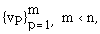be a maximal orthonormal sequence in the nullspace of A*. We know there is G( ,p) in Rn such that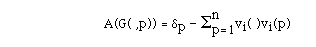for < dp- [[Sigma]]i=1vi( ) vi(p) , w > = 0

for all w in the nullspace of A*. We will show that u(i) defined by

< G(i, ), f >

satisfies the equation Au = f. In fact,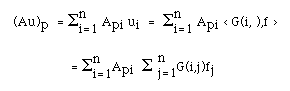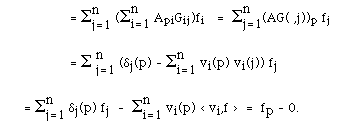Exercises A.1.    (a) Suppose that L: R3 -> R and is defined by L(u) = 2u1-u3. Find v such that L(u) = < u, v > for all u on R3.

(b) Suppose that L: R3 -> R and is defined by L(u)= u2. Find v such that L(u) = < u, v >.

(c) Give the matrix representation of L: R3 -> R3 if L(u) = {3u1+2u3, -u1+u2, u1-u2+u3}.

A.2.    (a)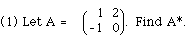(b) For the following matrices A and vectors f, determing whether the equation Au = f has exactly one solution, no solution or an infinity of solutions. If there are solutions, find them.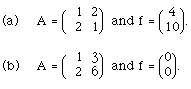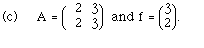A.3.    (a) For each of the matrices listed below determine what is the dimension of the null space of A and of the null space of A*? Give a basis for each. Find one f such that < f, z > = 0 for each z in the null space of A*. Solve Au = f. Find g such that < g, z > != 0 for each z in the null space of A*. Show that one can not solve Au=g for this g.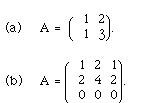(b) Let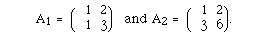Show that the problem A1 u = f is well posed and the problem A2 u = f is not.

A.4.    (a) For the following matrices A, show that det(A) = 0, Find an orthonormal basis for the null space of A*. Make up G. For the given f show that is it is perpendicular to the null space of A*. Show that u as defined by equation (*) in the discussion of the First Alternative solves Au=f.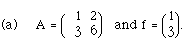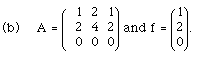(2) Take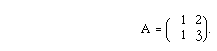Find G such that the equation

< G(i .), f(.) > = u(i)

provides a solution for Au = f for any vector f. ( Be aware that high school students know how to do this without ever thinking of the vector deltai!)

Back to Chapter XVIII